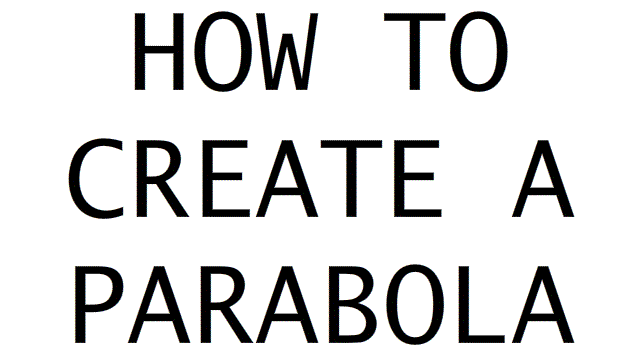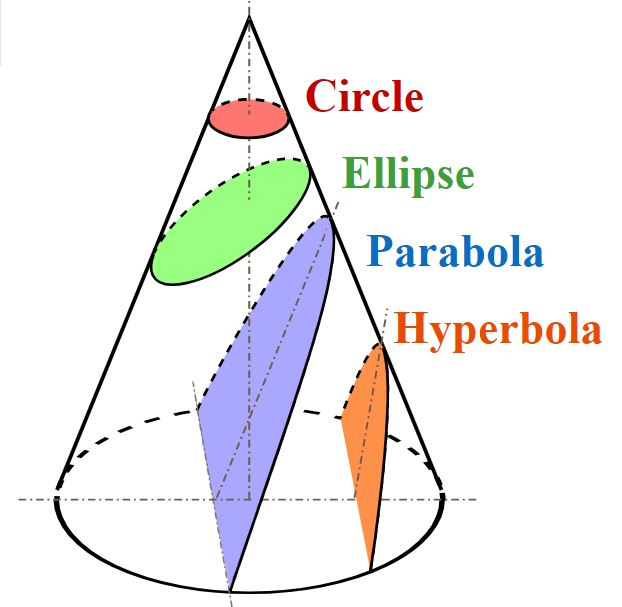# Formation of Circle, Ellipse, Parabola and Hyperbola from Cone

## Parabola

A parabola is the locus of points that is equidistant from both a certain point (called the focus) and a certain line (called the directrix). The parabola is the conic section that is obtained by slicing the cone parallel to the edge of the cone.Fig: Creating a ParabolaTHE ELLIPSE

The section is an ellipse when a plane is inclined to the axis and cuts all the generators.( a generator is a straight line which runs from the apex of the cone to the base )THE PARABOLA

The section is a parabola when the plane is parallel to a generator, and only one of the cone.THE HYPERBOLA

The section gives a hyperbola when the cutting plane is inclined at an angle greater than the exterior angle of the cone.http://www3.ul.ie/~rynnet/swconics/planes_cutting_coneA.htm